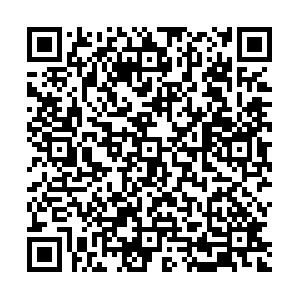## 留言板引用本文: 刘久富, 张信哲, 汪恒宇, 等 . 部分可观Petri网故障的量子贝叶斯诊断[J]. 北京航空航天大学学报, 2022, 48(7): 1125-1134.LIU Jiufu, ZHANG Xinzhe, WANG Hengyu, et al. Partial observable Petri nets fault diagnosis with quantum Bayesian learning[J]. Journal of Beijing University of Aeronautics and Astronautics, 2022, 48(7): 1125-1134. doi: 10.13700/j.bh.1001-5965.2021.0010(in Chinese)
 Citation: LIU Jiufu, ZHANG Xinzhe, WANG Hengyu, et al. Partial observable Petri nets fault diagnosis with quantum Bayesian learning[J]. Journal of Beijing University of Aeronautics and Astronautics, 2022, 48(7): 1125-1134. (in Chinese)• 中图分类号: TP391

## Partial observable Petri nets fault diagnosis with quantum Bayesian learning

Funds:

National Natural Science Foundation of China 61473144

###### Corresponding author:LIU Jiufu, E-mail: liujiufu2@126.com
• 摘要:

针对故障本身构建量子贝叶斯Petri网模型算法，并利用该子网模型进行Petri网系统故障分析。对于部分可观Petri网模型中的不可观故障，根据可达标识图分析变迁点火路径不能判断系统状态，建立量子贝叶斯子网模型，通过不确定路径引起的量子干涉重新标定变迁的条件概率表得到量子概率振幅表。根据故障变迁的前置集合并结合量子贝叶斯推理计算变迁触发的先验概率，由后置集合中的可观变迁修正后验概率，由最大后验概率估计系统所处状态，当故障变迁不唯一时，选取最大概率的故障作为故障源。以实际故障系统建立部分可观Petri网模型，结合可观标签概率序列信息和量子贝叶斯概率估计，对系统不可观部分进行故障诊断验证算法的有效性。

• 图 1  离散事件动态系统的POPN模型

Figure 1.  POPN model of discrete event dynamic system

图 2  故障变迁t10对应的QBPN模型

Figure 2.  QBPN model of fault transition t10

图 3  量子干涉影响的变迁t9

Figure 3.  Transition t9 of the influence of quantum interference

图 4  量子干涉影响的变迁t14

Figure 4.  Transition t14 of the influence of quantum interference

图 5  量子干涉影响的变迁t15

Figure 5.  Transition t15 of the influence of quantum interference

图 6  经典贝叶斯和量子贝叶斯概率

Figure 6.  Classical Bayesian probability and quantum Bayesian probability

图 7  t5条件下t14的量子贝叶斯概率值变化曲线

Figure 7.  Change of quantum Bayesian probability value of t14 under the condition of t5

图 8  t5条件下的量子贝叶斯概率值变化曲线

Figure 8.  Change of quantum Bayesian probability value ofunder the condition of t5

图 9  由变迁t3t8的不确定路径

Figure 9.  Uncertain path from transition t3 to t8

图 10  干涉角{θ1, θ2}引起t3条件下t8点火概率变化

Figure 10.  Interference angle {θ1, θ2} causes the change of firing probability of t8 under the condition of t3

图 11  干涉角{θ3, θ4}引起t3条件下t8点火概率变化

Figure 11.  Interference angle {θ3, θ4} causes the change of firing probability of t8 under the condition of t3

图 12  基于BPN算法的概率分布

Figure 12.  Probability distribution based on BPN algorithm

图 13  基于QBPN算法的概率分布

Figure 13.  Probability distribution based on QBPN algorithm

图 14  BPN算法和QBPN算法故障诊断概率比较

Figure 14.  Comparison of fault diagnosis probability between BPN algorithm and QBPN algorithm

表  1  总概率情况和量子贝叶斯情况的概率估计比较

Table  1.   Comparison of probability estimates between total probability and quantum Bayesian

 概率估计 概率值 Pr(t8|t5) 0.81 φ(t8|t5)Pr(t10|t5) 0.19 φ(t10|t5)Pr(t14|t8) 0.63 φ(t14|t8)Pr(t14|t10) 0.43 φ(t14|t10)总概率下Pr(t14|t5) 0.592 量子贝叶斯Pr(t14|t5) 0.795 5 总概率下Pr(t14|t5) 0.408 量子贝叶斯Pr(t14|t5) 0.204 5

表  2  基于POPN的量子贝叶斯故障算法故障诊断结果

Table  2.   Fault diagnosis results of quantum Bayesian fault algorithm based on POPN

 可观概率序列σo 故障变迁Tfi触发概率 干涉角θ/rad QB函数结果 置信度Fb 系统故障概率Pr(σo, ψ, Tfi) 系统状态估计W 系统实际状态 (t0, 1)(t4, 0.883)(t3, 0.926) Pr(tf10|t0, t4, t3) π/2 0 0 0 N 无故障 (t0, 1)(t4, 0.883) (t3, 0.926)(t15, 0.879) Pr(tf10|t0, t4, t3) π/2 0.162 1 0.4 0.047 1 N 无故障 Pr(tf10|t0, t4, t3, t15) 0 0.047 1 Pr(tf11|t0, t4, t3) π/2 0.158 6 Pr(tf11|t0, t4, t3, t15) 0 0.007 6 (t0, 1)(t4, 0.883) (t3, 0.926)(t14, 0.851) (t15, 0.879)(t12, 0.463) Pr(tf10|t0, t4, t3) π/2 0.162 1 0.6 0.966 8 F 故障 Pr(tf10|t0, t4, t3, t14) 0 0.756 9 Pr(tf10|t0, t4, t3, t14, t15) 0 0.926 3 Pr(tf10|t0, t4, t3, t14, t15, t12) 0 0.966 8 Pr(tf11|t0, t4, t3) π/2 0.158 6 Pr(tf11|t0, t4, t3, t14, t15, t12) 0 0.000 6 (t0, 1)(t3, 0.907) (t13, 0.736) Pr(tf11|t0, t3, t13) π/2 1 1 1 F 故障

表  3  QBPN算法与BPN算法比较

Table  3.   Comparison of QBPN algorithm and BPN algorithm

 算法 响应时间/ms 诊断正确率/% BPN 300 93.70 QBPN 230 98.74
•点击查看大图
##### 计量
• 文章访问数:  41
• HTML全文浏览量:  12
• PDF下载量:  9
• 被引次数: 0
##### 出版历程
• 收稿日期:  2021-01-08
• 录用日期:  2021-03-20
• 刊出日期:  2021-05-06

### 目录/下载:  全尺寸图片 幻灯片
• 分享
• 用微信扫码二维码

分享至好友和朋友圈

常见问答